# Krylov-Bogolyubov method of averaging

A method used in non-linear oscillation theory to study oscillatory processes; it is based on an averaging principle, that is, the exact differential equation of the motion is replaced by an averaged equation.

Long before the work of N.M. Krylov and N.N. Bogolyubov, various averaging schemes (Gauss, Fatou, Delone–Hill, etc.) were widely applied in celestial mechanics. These two authors have the credit of working out a general algorithm, known as the Krylov–Bogolyubov method of averaging, and proving that the solutions of the averaged system approximate those of the exact one (see , ). The rigorous theory of the method, with a comprehensive explanation of the essence of the general averaging principle, is due to N.N. Bogolyubov (see , ), who showed that the averaging method is related to the existence of a certain transformation of variables which enables one to eliminate the timefrom the free terms of the equations, up to a given degree of accuracy in terms of a small parameter; he also established the asymptotic nature of the approximations that the method yield, and established a relationship between the solutions of the exact and the averaged equations over an infinite time interval. These results were later extended by Yu.A. Mitropol'skii and others (see ); they are used in the study of non-linear oscillations.

The standard form of the system of equations for which the Krylov–Bogolyubov method of averaging has been developed is:(1)

whereis the time andis a small positive parameter. The fundamental assumptions adopted in regard to this system are thatis a smooth function of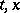and that this function is in a sense "recurrent" in, implying the existence of the averagee.g.might be a periodic or almost-periodic function of.

The-th approximation to the solutionof the system (1) is, according to the method, defined by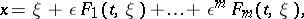(2)

where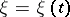is the solution of the "averaged" equation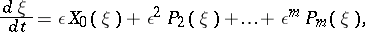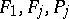,, are functions chosen so that the expression (2) should satisfy equation (1) up to quantities of orderand so that the functionsshould satisfy the same recurrence conditions inas the free term of equation (1). The determination of the functionsis elementary; the functionsare found by averaging the right-hand side of equation (1) in whichhas been replaced by (2). In particular, ifis a periodic function of, with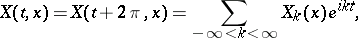(3)

the function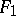is determined from (3) byand the functions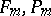() are determined by analogous formulas using the relation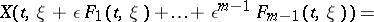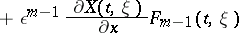The validity of the averaging method is established as follows. 1) One proves an estimate of the typewhere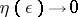as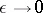,is a constant independent of, and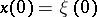; 2) one proves the existence of a solution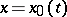of the system (1) which lies in a sufficiently small neighbourhood of the equilibrium position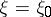,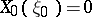, of the averaged system: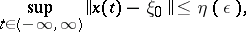and shows that this solution is stable and periodic or almost-periodic; 3) one proves the existence of an integral manifold:of the system (1), in a neighbourhood of a periodic trajectory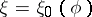,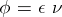,, of the averaged system: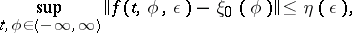and investigates the behaviour of the solutions of (1) that lie in the neighbourhood of the manifold.